Definition: a Quadratic function is called a function of the form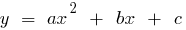where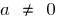1. Scope2.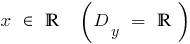3. Multiple value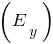4. when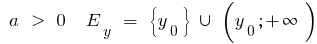when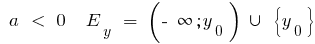5. Parity, odd parity
6. ifthe function is neither even nor odd

in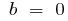— steamroom

7. Continuity and differentiability
8. A quadratic function is continuous and diferencian on the entire number line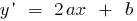9. The increase and decrease, extrema
10. if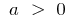is decreasing on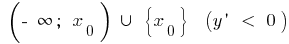and increasing on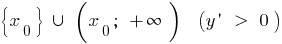,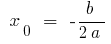— minimum,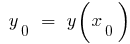— low

when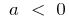increasing onand decreasing on,— high,— high

11. The graph of a quadratic function is always a parabolawhose branches are directed upwards whenand down when12. Coordinates of the vertex of the parabola:;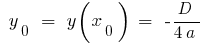where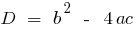The axis of symmetry of a parabola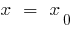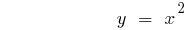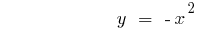Symmetry relative to the axis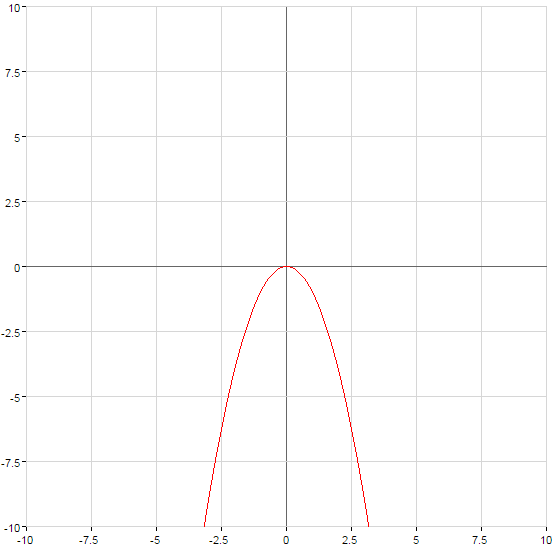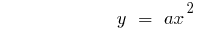The graph of the function is stretched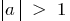or stretched with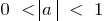respect to the axisby the number of units equal to the number and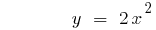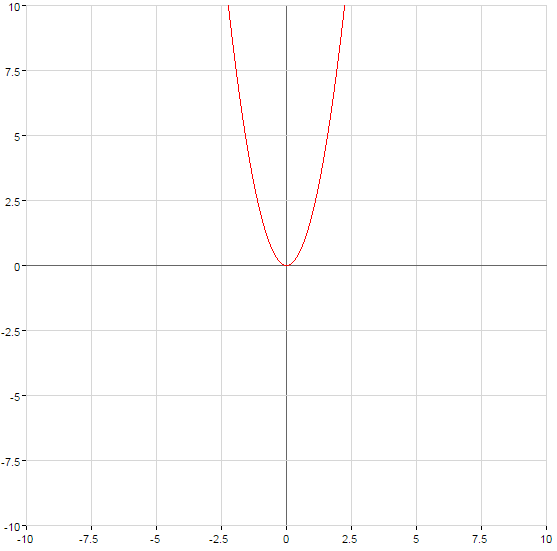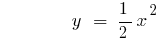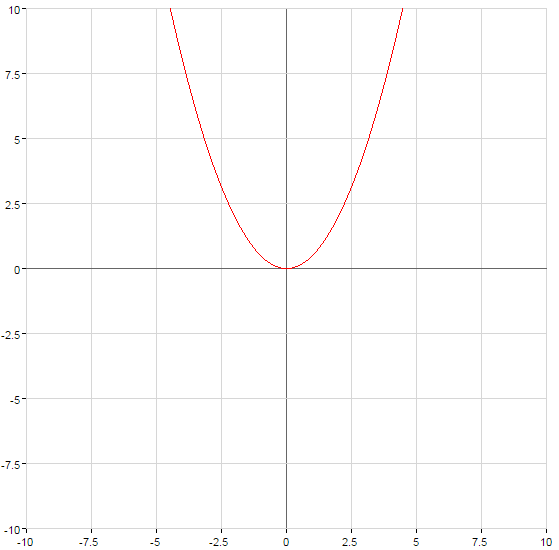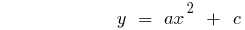The graph of the function rises at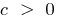or falls with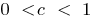the number of units equal to the number of c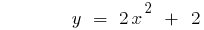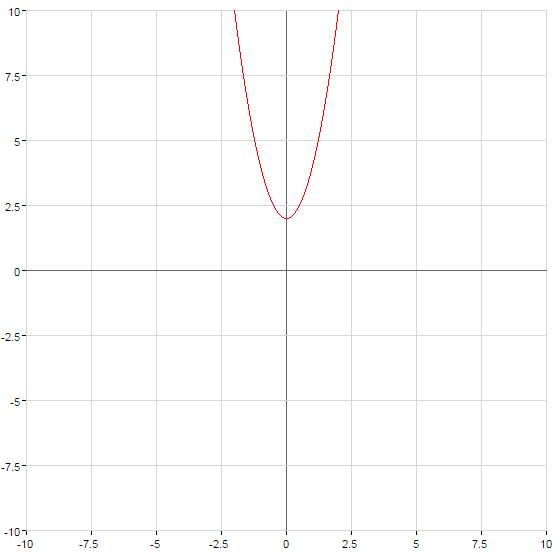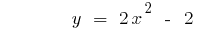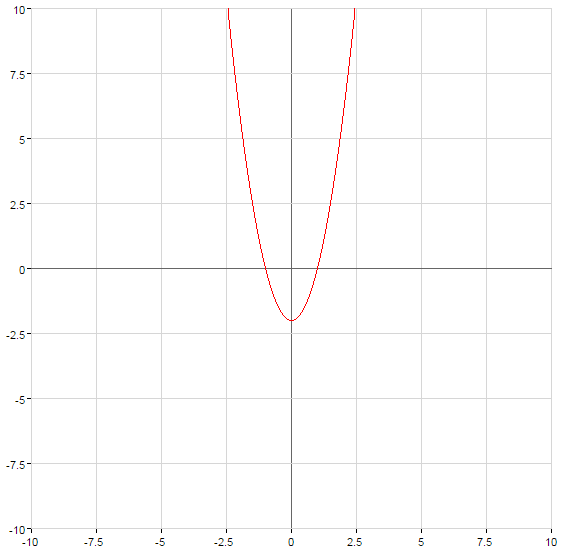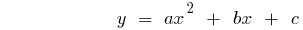The parabola crosses the axis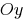at the point C## How to graph quadratic functions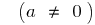And method

1. To calculate abscissa tops
2.3. Substituteinto the equation and calculate the y coordinate of the top —4. To build a sketch of the parabola (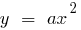) vertex at point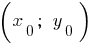5. whenthe branches up, with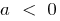the branches down

II method

2. Using the elementary transformations of graphs, to perform a parallel transfer of a parabola3. (along the axison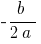, axison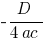)